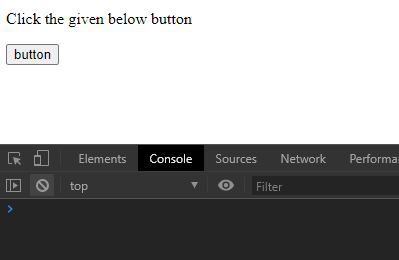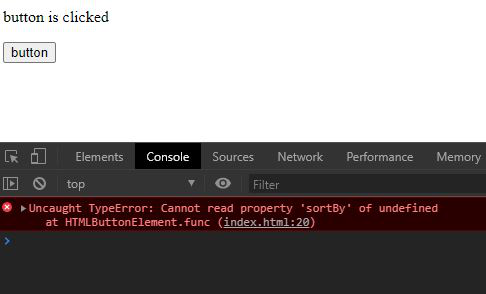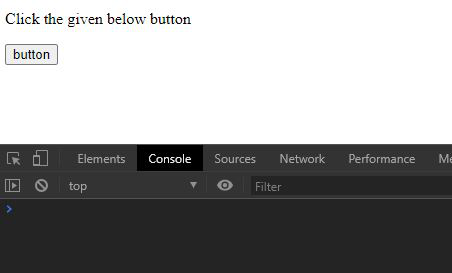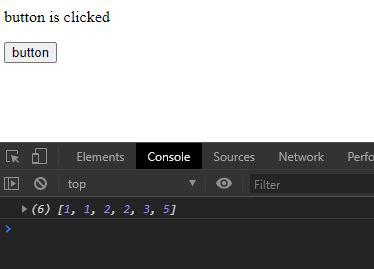# Underscore.js _.noConflict() Function

• Last Updated : 28 Oct, 2021

Underscore.js is a library in javascript that makes operations on arrays, string, objects much easier and handy.
The _.noConflict() function is used to create a reference of the global underscore object “_” to another variable.

Note: It is very necessary to link the underscore CDN before going and using underscore functions in the browser. When linking the underscore.js CDN The “_” is attached to the browser as a global variable.

Syntax:

`_.noConflict()`

Parameters: This function does not accept any parameter.

Return Value: It returns the reference to the global underscore variable.

Example 1: When noConflict() function is not used and using “underscore” variable.

## HTML

 ```<``html``>` `<``head``>``    ``<``script` `src``=``"https://cdnjs.cloudflare.com/ajax/libs/underscore.js/1.9.1/underscore-min.js"``>``    ````` `<``body``>` `    ` `<``p``>Click the given below button`  `    ``<``button``>``        ``button``    ``` `    ``<``script``>``        ``let btn = document.querySelector("button");``        ``let p = document.querySelector("p")` `        ``// Creating a array``        ``let arr = [2, 3, 1, 2, 5, 1];` `        ``// Declaring underscore variable``        ``let underscore;` `        ``// Creating a function``        ``let func = () => {` `            ``// Changing text of paragraph``            ``// on button click``            ``p.innerText = "button is clicked";` `            ``// Sorting the array``            ``arr = underscore.sortBy(arr,``                ``(e) => { return Math.round(e) })``            ``console.log(arr)``        ``}``        ``btn.addEventListener("click", func);``    ````` ``

Output:

• When button is not clicked:• When button is clicked:Example 2: When noConflict() function is used.

## HTML

 ```<``html``>` `<``head``>``    ``<``script` `src``=``"https://cdnjs.cloudflare.com/ajax/libs/underscore.js/1.9.1/underscore-min.js"``>``    ````` `<``body``>` `    ` `<``p``>Click the given below button`  `    ``<``button``>``        ``button``    ``` `    ``<``script``>``        ``let btn = document.querySelector("button");``        ``let p = document.querySelector("p")``        ``let arr = [2, 3, 1, 2, 5, 1];` `        ``// Using underscore as a reference``        ``// to global _ variable``        ``let underscore = _.noConflict();` `        ``// Creating a function named func```        ``let func = () => {``            ``p.innerText = "button is clicked";``            ``arr = underscore.sortBy(arr,``                ``(e) => { return Math.round(e) })``            ``console.log(arr)``        ``}``        ` `        ``// Adding event listener to button``        ``btn.addEventListener("click", func);``    ````` ``

Output:

• When button is not clicked:• When button is clicked:My Personal Notes arrow_drop_up